Qrack  1.7 General classical-emulating-quantum development framework
Rotational gates:

NOTE: Dyadic operation angle sign is reversed from radian rotation operators and lacks a division by a factor of two. More...

## Functions

virtual void Qrack::QInterface::RT (real1 radians, bitLenInt qubitIndex)
Phase shift gate. More...

virtual void Qrack::QInterface::RTDyad (int numerator, int denomPower, bitLenInt qubitIndex)
Dyadic fraction phase shift gate. More...

virtual void Qrack::QInterface::RX (real1 radians, bitLenInt qubitIndex)
X axis rotation gate. More...

virtual void Qrack::QInterface::RXDyad (int numerator, int denomPower, bitLenInt qubitIndex)
Dyadic fraction X axis rotation gate. More...

virtual void Qrack::QInterface::Exp (real1 radians, bitLenInt qubitIndex)
(Identity) Exponentiation gate More...

virtual void Qrack::QInterface::ExpDyad (int numerator, int denomPower, bitLenInt qubitIndex)
Dyadic fraction (identity) exponentiation gate. More...

virtual void Qrack::QInterface::ExpX (real1 radians, bitLenInt qubitIndex)
Pauli X exponentiation gate. More...

virtual void Qrack::QInterface::ExpXDyad (int numerator, int denomPower, bitLenInt qubitIndex)
Dyadic fraction Pauli X exponentiation gate. More...

virtual void Qrack::QInterface::ExpY (real1 radians, bitLenInt qubitIndex)
Pauli Y exponentiation gate. More...

virtual void Qrack::QInterface::ExpYDyad (int numerator, int denomPower, bitLenInt qubitIndex)
Dyadic fraction Pauli Y exponentiation gate. More...

virtual void Qrack::QInterface::ExpZ (real1 radians, bitLenInt qubitIndex)
Pauli Z exponentiation gate. More...

virtual void Qrack::QInterface::ExpZDyad (int numerator, int denomPower, bitLenInt qubitIndex)
Dyadic fraction Pauli Z exponentiation gate. More...

virtual void Qrack::QInterface::CRX (real1 radians, bitLenInt control, bitLenInt target)
Controlled X axis rotation gate. More...

virtual void Qrack::QInterface::CRXDyad (int numerator, int denomPower, bitLenInt control, bitLenInt target)
Controlled dyadic fraction X axis rotation gate. More...

virtual void Qrack::QInterface::RY (real1 radians, bitLenInt qubitIndex)
Y axis rotation gate. More...

virtual void Qrack::QInterface::RYDyad (int numerator, int denomPower, bitLenInt qubitIndex)
Dyadic fraction Y axis rotation gate. More...

virtual void Qrack::QInterface::CRY (real1 radians, bitLenInt control, bitLenInt target)
Controlled Y axis rotation gate. More...

virtual void Qrack::QInterface::CRYDyad (int numerator, int denomPower, bitLenInt control, bitLenInt target)
Controlled dyadic fraction y axis rotation gate. More...

virtual void Qrack::QInterface::RZ (real1 radians, bitLenInt qubitIndex)
Z axis rotation gate. More...

virtual void Qrack::QInterface::RZDyad (int numerator, int denomPower, bitLenInt qubitIndex)
Dyadic fraction Z axis rotation gate. More...

virtual void Qrack::QInterface::CRZ (real1 radians, bitLenInt control, bitLenInt target)
Controlled Z axis rotation gate. More...

virtual void Qrack::QInterface::CRZDyad (int numerator, int denomPower, bitLenInt control, bitLenInt target)
Controlled dyadic fraction Z axis rotation gate. More...

virtual void Qrack::QInterface::CRT (real1 radians, bitLenInt control, bitLenInt target)
Controlled "phase shift gate". More...

virtual void Qrack::QInterface::CRTDyad (int numerator, int denomPower, bitLenInt control, bitLenInt target)
Controlled dyadic fraction "phase shift gate". More...

virtual void Qrack::QUnit::RT (real1 radians, bitLenInt qubit)
Phase shift gate. More...

virtual void Qrack::QUnit::RX (real1 radians, bitLenInt qubit)
X axis rotation gate. More...

virtual void Qrack::QUnit::RY (real1 radians, bitLenInt qubit)
Y axis rotation gate. More...

virtual void Qrack::QUnit::RZ (real1 radians, bitLenInt qubit)
Z axis rotation gate. More...

virtual void Qrack::QUnit::Exp (real1 radians, bitLenInt qubit)
(Identity) Exponentiation gate More...

virtual void Qrack::QUnit::ExpX (real1 radians, bitLenInt qubit)
Pauli X exponentiation gate. More...

virtual void Qrack::QUnit::ExpY (real1 radians, bitLenInt qubit)
Pauli Y exponentiation gate. More...

virtual void Qrack::QUnit::ExpZ (real1 radians, bitLenInt qubit)
Pauli Z exponentiation gate. More...

virtual void Qrack::QUnit::CRX (real1 radians, bitLenInt control, bitLenInt target)
Controlled X axis rotation gate. More...

virtual void Qrack::QUnit::CRY (real1 radians, bitLenInt control, bitLenInt target)
Controlled Y axis rotation gate. More...

virtual void Qrack::QUnit::CRZ (real1 radians, bitLenInt control, bitLenInt target)
Controlled Z axis rotation gate. More...

virtual void Qrack::QUnit::CRT (real1 radians, bitLenInt control, bitLenInt target)
Controlled "phase shift gate". More...

## Detailed Description

NOTE: Dyadic operation angle sign is reversed from radian rotation operators and lacks a division by a factor of two.

## Function Documentation

 void Qrack::QUnit::CRT ( real1 radians, bitLenInt control, bitLenInt target )
virtual

Controlled "phase shift gate".

Controlled "phase shift gate" - if control bit is true, rotates target bit as e^(-i*/2) around |1> state.

If control bit is set to 1, rotates target bit as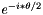around |1> state.

Reimplemented from Qrack::QInterface.

 void Qrack::QInterface::CRT ( real1 radians, bitLenInt control, bitLenInt target )
virtual

Controlled "phase shift gate".

Controlled "phase shift gate" - if control bit is true, rotates target bit as e^(-i*/2) around |1> state.

If control bit is set to 1, rotates target bit asaround |1> state.

Reimplemented in Qrack::QUnit, and Qrack::QEngineOCLMulti.

 void Qrack::QInterface::CRTDyad ( int numerator, int denomPower, bitLenInt control, bitLenInt target )
virtual

Controlled dyadic fraction "phase shift gate".

Controlled dyadic "phase shift gate" - if control bit is true, rotates target bit as e^(i*(M_PI * numerator) / 2^denomPower) around |1> state.

If control bit is set to 1, rotates target bit as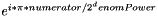around |1> state.

 void Qrack::QUnit::CRX ( real1 radians, bitLenInt control, bitLenInt target )
virtual

Controlled X axis rotation gate.

Controlled x axis rotation - if control bit is true, rotates as e^(-i*/2) around Pauli x axis.

If "control" is 1, rotates ason Pauli x axis.

Reimplemented from Qrack::QInterface.

 void Qrack::QInterface::CRX ( real1 radians, bitLenInt control, bitLenInt target )
virtual

Controlled X axis rotation gate.

Controlled x axis rotation - if control bit is true, rotates as e^(-i*/2) around Pauli x axis.

If "control" is 1, rotates ason Pauli x axis.

Reimplemented in Qrack::QUnit, and Qrack::QEngineOCLMulti.

 void Qrack::QInterface::CRXDyad ( int numerator, int denomPower, bitLenInt control, bitLenInt target )
virtual

Controlled dyadic fraction X axis rotation gate.

Controlled dyadic fraction x axis rotation gate - Rotates as e^(i*(M_PI * numerator) / 2^denomPower) around Pauli x axis.

If "control" is 1, rotates asaround Pauli x axis.

NOTE THAT DYADIC OPERATION ANGLE SIGN IS REVERSED FROM RADIAN ROTATION OPERATORS.

 void Qrack::QUnit::CRY ( real1 radians, bitLenInt control, bitLenInt target )
virtual

Controlled Y axis rotation gate.

Controlled y axis rotation - if control bit is true, rotates as e^(-i*) around Pauli y axis.

If "control" is set to 1, rotates asaround Pauli Y axis.

Reimplemented from Qrack::QInterface.

 void Qrack::QInterface::CRY ( real1 radians, bitLenInt control, bitLenInt target )
virtual

Controlled Y axis rotation gate.

Controlled y axis rotation - if control bit is true, rotates as e^(-i*) around Pauli y axis.

If "control" is set to 1, rotates asaround Pauli Y axis.

Reimplemented in Qrack::QUnit, and Qrack::QEngineOCLMulti.

 void Qrack::QInterface::CRYDyad ( int numerator, int denomPower, bitLenInt control, bitLenInt target )
virtual

Controlled dyadic fraction y axis rotation gate.

Controlled dyadic fraction y axis rotation gate - Rotates as e^(i*(M_PI * numerator) / 2^denomPower) around Pauli y axis.

If "control" is set to 1, rotates asaround Pauli Y axis.

NOTE THAT DYADIC OPERATION ANGLE SIGN IS REVERSED FROM RADIAN ROTATION OPERATORS.

 void Qrack::QUnit::CRZ ( real1 radians, bitLenInt control, bitLenInt target )
virtual

Controlled Z axis rotation gate.

Controlled z axis rotation - if control bit is true, rotates as e^(-i*) around Pauli z axis.

If "control" is set to 1, rotates asaround Pauli Zaxis.

Reimplemented from Qrack::QInterface.

 void Qrack::QInterface::CRZ ( real1 radians, bitLenInt control, bitLenInt target )
virtual

Controlled Z axis rotation gate.

Controlled z axis rotation - if control bit is true, rotates as e^(-i*) around Pauli z axis.

If "control" is set to 1, rotates asaround Pauli Zaxis.

Reimplemented in Qrack::QUnit, and Qrack::QEngineOCLMulti.

 void Qrack::QInterface::CRZDyad ( int numerator, int denomPower, bitLenInt control, bitLenInt target )
virtual

Controlled dyadic fraction Z axis rotation gate.

Controlled dyadic fraction z axis rotation gate - Rotates as e^(i*(M_PI * numerator) / 2^denomPower) around Pauli z axis.

If "control" is set to 1, rotates asaround Pauli Z axis.

NOTE THAT DYADIC OPERATION ANGLE SIGN IS REVERSED FROM RADIAN ROTATION OPERATORS.

 void Qrack::QUnit::Exp ( real1 radians, bitLenInt qubitIndex )
virtual

(Identity) Exponentiation gate

Exponentiate identity operator.

Applies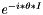, exponentiation of the identity operator

Reimplemented from Qrack::QInterface.

 void Qrack::QInterface::Exp ( real1 radians, bitLenInt qubitIndex )
virtual

(Identity) Exponentiation gate

Exponentiate identity operator.

Applies, exponentiation of the identity operator

Reimplemented in Qrack::QUnit, and Qrack::QEngineOCLMulti.

 void Qrack::QInterface::ExpDyad ( int numerator, int denomPower, bitLenInt qubit )
virtual

Dyadic fraction (identity) exponentiation gate - Applies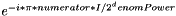, exponentiation of the identity operator.

Applies, exponentiation of the identity operator

NOTE THAT DYADIC OPERATION ANGLE SIGN IS REVERSED FROM RADIAN ROTATION OPERATORS AND LACKS DIVISION BY A FACTOR OF TWO.

 void Qrack::QUnit::ExpX ( real1 radians, bitLenInt qubitIndex )
virtual

Pauli X exponentiation gate.

Exponentiate Pauli X operator.

Applies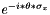, exponentiation of the Pauli X operator

Reimplemented from Qrack::QInterface.

 void Qrack::QInterface::ExpX ( real1 radians, bitLenInt qubitIndex )
virtual

Pauli X exponentiation gate.

Exponentiate Pauli X operator.

Applies, exponentiation of the Pauli X operator

Reimplemented in Qrack::QUnit, and Qrack::QEngineOCLMulti.

 void Qrack::QInterface::ExpXDyad ( int numerator, int denomPower, bitLenInt qubit )
virtual

Dyadic fraction Pauli X exponentiation gate.

Dyadic fraction Pauli X exponentiation gate - Applies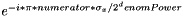, exponentiation of the Pauli X operator.

Applies, exponentiation of the Pauli X operator

NOTE THAT DYADIC OPERATION ANGLE SIGN IS REVERSED FROM RADIAN ROTATION OPERATORS AND LACKS DIVISION BY A FACTOR OF TWO.

 void Qrack::QUnit::ExpY ( real1 radians, bitLenInt qubitIndex )
virtual

Pauli Y exponentiation gate.

Exponentiate Pauli Y operator.

Applies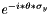, exponentiation of the Pauli Y operator

Reimplemented from Qrack::QInterface.

 void Qrack::QInterface::ExpY ( real1 radians, bitLenInt qubitIndex )
virtual

Pauli Y exponentiation gate.

Exponentiate Pauli Y operator.

Applies, exponentiation of the Pauli Y operator

Reimplemented in Qrack::QUnit, and Qrack::QEngineOCLMulti.

 void Qrack::QInterface::ExpYDyad ( int numerator, int denomPower, bitLenInt qubit )
virtual

Dyadic fraction Pauli Y exponentiation gate.

Dyadic fraction Pauli Y exponentiation gate - Applies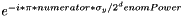, exponentiation of the Pauli Y operator.

Applies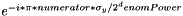, exponentiation of the Pauli Y operator

NOTE THAT DYADIC OPERATION ANGLE SIGN IS REVERSED FROM RADIAN ROTATION OPERATORS AND LACKS DIVISION BY A FACTOR OF TWO.

 void Qrack::QUnit::ExpZ ( real1 radians, bitLenInt qubitIndex )
virtual

Pauli Z exponentiation gate.

Exponentiate Pauli Z operator.

Applies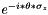, exponentiation of the Pauli Z operator

Reimplemented from Qrack::QInterface.

 void Qrack::QInterface::ExpZ ( real1 radians, bitLenInt qubitIndex )
virtual

Pauli Z exponentiation gate.

Exponentiate Pauli Z operator.

Applies, exponentiation of the Pauli Z operator

Reimplemented in Qrack::QUnit, and Qrack::QEngineOCLMulti.

 void Qrack::QInterface::ExpZDyad ( int numerator, int denomPower, bitLenInt qubit )
virtual

Dyadic fraction Pauli Z exponentiation gate.

Dyadic fraction Pauli Z exponentiation gate - Applies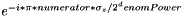, exponentiation of the Pauli Z operator.

Applies, exponentiation of the Pauli Z operator

NOTE THAT DYADIC OPERATION ANGLE SIGN IS REVERSED FROM RADIAN ROTATION OPERATORS AND LACKS DIVISION BY A FACTOR OF TWO.

 void Qrack::QUnit::RT ( real1 radians, bitLenInt qubitIndex )
virtual

Phase shift gate.

"Phase shift gate" - Rotates as e^(-i*/2) around |1> state

Rotates asaround |1> state

Reimplemented from Qrack::QInterface.

 void Qrack::QInterface::RT ( real1 radians, bitLenInt qubitIndex )
virtual

Phase shift gate.

"Phase shift gate" - Rotates as e^(-i*/2) around |1> state

Rotates asaround |1> state

Reimplemented in Qrack::QUnit, and Qrack::QEngineOCLMulti.

 void Qrack::QInterface::RTDyad ( int numerator, int denomPower, bitLenInt qubit )
virtual

Dyadic fraction "phase shift gate" - Rotates as e^(i*(M_PI * numerator) / 2^denomPower) around |1> state.

Rotates asaround |1> state.

NOTE THAT * DYADIC OPERATION ANGLE SIGN IS REVERSED FROM RADIAN ROTATION OPERATORS AND LACKS DIVISION BY A FACTOR OF TWO.

 void Qrack::QUnit::RX ( real1 radians, bitLenInt qubitIndex )
virtual

X axis rotation gate.

x axis rotation gate - Rotates as e^(-i*/2) around Pauli x axis

Rotates asaround Pauli X axis

Reimplemented from Qrack::QInterface.

 void Qrack::QInterface::RX ( real1 radians, bitLenInt qubitIndex )
virtual

X axis rotation gate.

x axis rotation gate - Rotates as e^(-i*/2) around Pauli x axis

Rotates asaround Pauli X axis

Reimplemented in Qrack::QUnit, and Qrack::QEngineOCLMulti.

 void Qrack::QInterface::RXDyad ( int numerator, int denomPower, bitLenInt qubit )
virtual

Dyadic fraction X axis rotation gate.

Dyadic fraction x axis rotation gate - Rotates as e^(i*(M_PI * numerator) / 2^denomPower) around Pauli x axis.

Rotateson Pauli x axis.

NOTE THAT DYADIC OPERATION ANGLE SIGN IS REVERSED FROM RADIAN ROTATION OPERATORS AND LACKS DIVISION BY A FACTOR OF TWO.

 void Qrack::QUnit::RY ( real1 radians, bitLenInt qubitIndex )
virtual

Y axis rotation gate.

y axis rotation gate - Rotates as e^(-i*/2) around Pauli y axis

Rotates asaround Pauli y axis.

Reimplemented from Qrack::QInterface.

 void Qrack::QInterface::RY ( real1 radians, bitLenInt qubitIndex )
virtual

Y axis rotation gate.

y axis rotation gate - Rotates as e^(-i*/2) around Pauli y axis

Rotates asaround Pauli y axis.

Reimplemented in Qrack::QUnit, and Qrack::QEngineOCLMulti.

 void Qrack::QInterface::RYDyad ( int numerator, int denomPower, bitLenInt qubit )
virtual

Dyadic fraction Y axis rotation gate.

Dyadic fraction y axis rotation gate - Rotates as e^(i*(M_PI * numerator) / 2^denomPower) around Pauli y axis.

Rotates asaround Pauli Y axis.

NOTE THAT DYADIC OPERATION ANGLE SIGN IS REVERSED FROM RADIAN ROTATION OPERATORS AND LACKS DIVISION BY A FACTOR OF TWO.

 void Qrack::QUnit::RZ ( real1 radians, bitLenInt qubitIndex )
virtual

Z axis rotation gate.

z axis rotation gate - Rotates as e^(-i*/2) around Pauli z axis

Rotates asaround Pauli Z axis.

Reimplemented from Qrack::QInterface.

 void Qrack::QInterface::RZ ( real1 radians, bitLenInt qubitIndex )
virtual

Z axis rotation gate.

z axis rotation gate - Rotates as e^(-i*/2) around Pauli z axis

Rotates asaround Pauli Z axis.

Reimplemented in Qrack::QUnit, and Qrack::QEngineOCLMulti.

 void Qrack::QInterface::RZDyad ( int numerator, int denomPower, bitLenInt qubit )
virtual

Dyadic fraction Z axis rotation gate.

Dyadic fraction y axis rotation gate - Rotates as e^(i*(M_PI * numerator) / 2^denomPower) around Pauli y axis.

Rotates asaround Pauli Z axis.

NOTE THAT DYADIC OPERATION ANGLE SIGN IS REVERSED FROM RADIAN ROTATION OPERATORS AND LACKS DIVISION BY A FACTOR OF TWO.